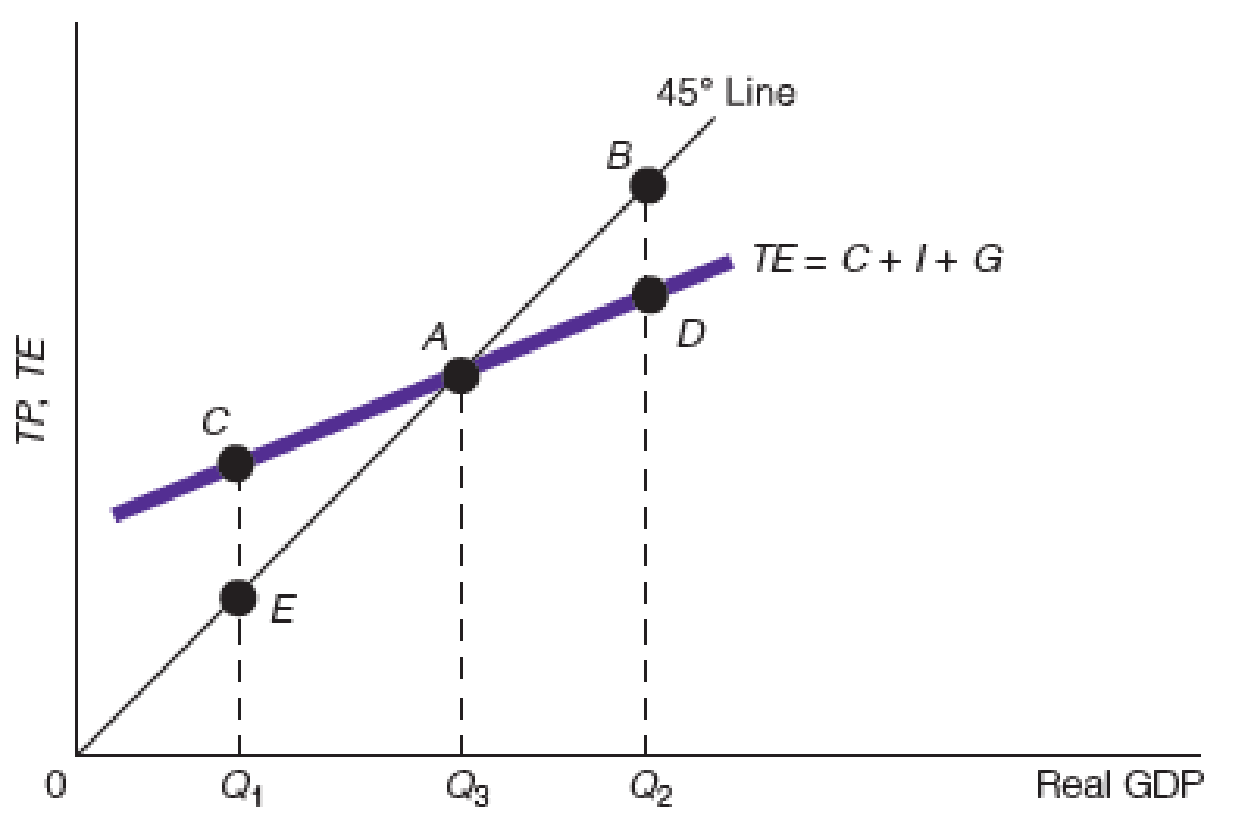Chapter 10, Problem 8WNG

Chapter
Section
Textbook Problem

In the accompanying figure, if Natural Real GDP is Q2, in what state is the economy at point A?To determine

The state of the economy when the economy is presently at Q2.

Explanation

The expenditures are of three types in the closed economy, namely the consumption expenditure, investment expenditure and the government purchases. These three expenditures in the economy are the expenditures that constitute the total expenditure of the economy. Thus, the total expenditure is the vertical summation of these three different expenditures of the economy. The TE of the economy is given as follows:

When the economy is at Q3, the TP and the TE are equal and there is no excess demand or production in the economy...

Still sussing out bartleby?

Check out a sample textbook solution.

See a sample solution

The Solution to Your Study Problems

Bartleby provides explanations to thousands of textbook problems written by our experts, many with advanced degrees!

Get Started